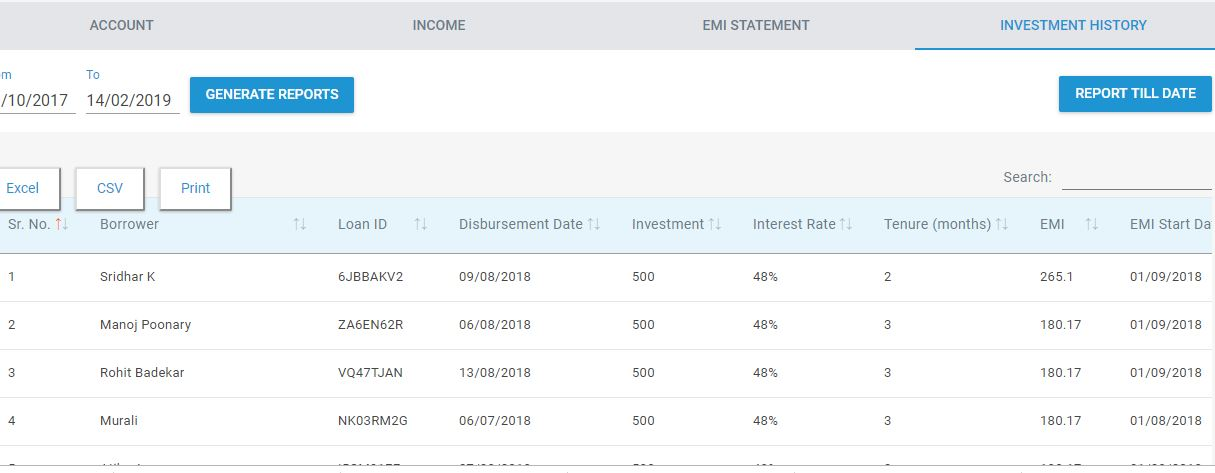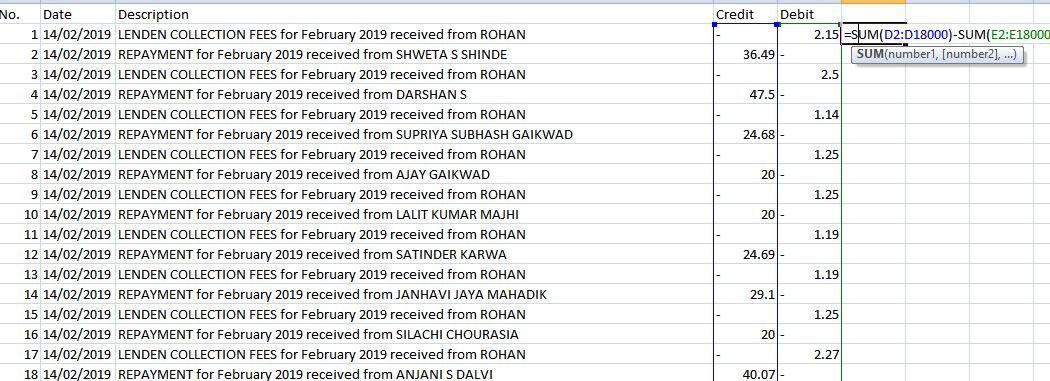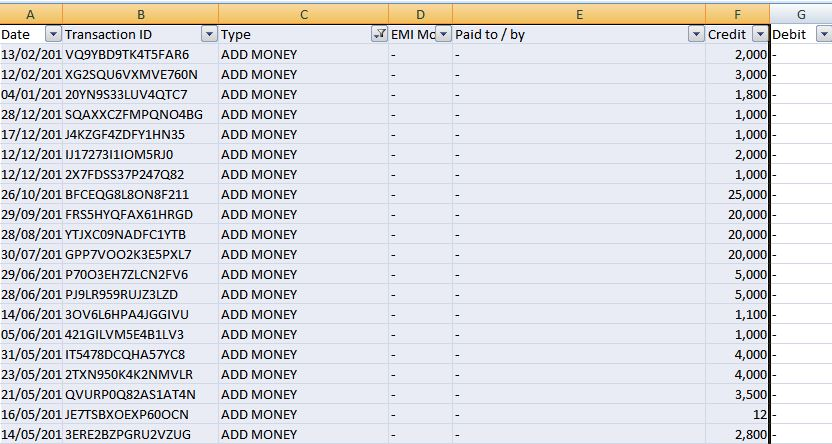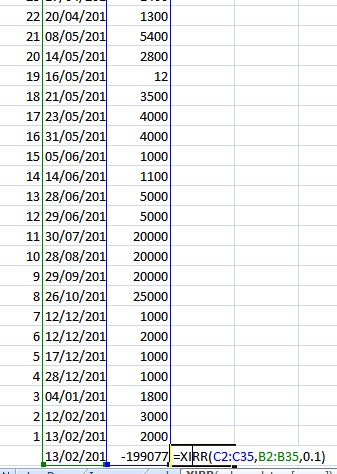# Calculating Portfolio Return for LendenClub

In my endeavour to create a robust P2P lending portfolio I will cover how I calculate return for my portfolio.I will start with LendenClub and in  the next  few posts will cover rest of the platforms.

The return provided on the platform are expected returns and can be misleading.That is a futuristic projection based on your loan investment and does not factor in the existing as well as potential NPA.

We need to calculate the Return on existing payments and NPA  of the portfolio.

2 method of calculating Returns are :

• Net Annualized rate of Return( NARR)
• Internal Rate of Return (XIRR)

Net Annualized Rate of Return is basically the Principal weighted rate of return. You put different amount in investment different months .Each Month you get different return. You calculate each month how much return you generate and what was the principal deployed and then you take the weighted average return

Problem is that this method does not  takes into consideration the amount of cash lying idle in the escrow amount not fetching any return. I will suggest using XIRR as we will find out it is a better approach.

XIRR : This method is fairly simple. It takes as input all the cashflow with the dates and the final amount achieved. One rate of return is calculated which will give us  that output based on the cashflow. As it takes our invested money ,cash drag if any due to escrow amount idle money is also factored in.

Now we know the method to calculate the return. One more thing we need to factor in is the NPA. We will deduct the NPA and   loans in delay  from the final result and then calculate the returns.

I use R programming language to calculate returns as it is fast and gives me more analysis capabilities like Future EMI projections etc

I will provide steps to calculate XIRR for lendenclub using excel as everybody is familiar with it.

Step1: Open Account section of LendenClub. Download the following  report from the date when you started investment.

1. Account
2. Income
3. Investment HistoryStep2: Income Statement Sheet

Difference between the two is the net earning.Step3: Investment History Sheet

Filter all the default ,written off and Delay 3 loans. Sum the principal outstanding. This is your potential default. Remember this is a conservative figure because some of the delay 3 loans will  be repayed but we want to take a conservative approach.Step4:Account

Filter all the “AddMoney” entries. this the money you have transferred from your account to Lenden.Select 5 :Calculation

Your Final Account value is  sum of (Addmoney) + Interest earning – defaults

i.e. You add all the rows of add money then take the net earning you got from income sheet and subtract the default you calculated from investment history.

Now add the final amount as last row after all the add money rows. You need to invert the add money series also ( you may need to convert dates from text to date format. Just use Left function in excel(Left(date entry,11), this will remove the extra part after date. For inverting series add a number series before the other columns and reorder it from large to small while  selecting “expand selection”.Now use XIRR ( make final amount as -ve). In XIRR you have need to put date series, cash series and a guess number (put naything 10% etc). You will get your XIRR number.

This is your portfolio return based on the conservative NPA assumption.

Points to Note:

• Calculate return after 4th of 5th day  of month after which date you generally receive bulk of EMI .
• I will calculate Return for other platform in a similar manner
• Total portfolio return is average  returns based  portfolio weights.

•  RupeeCircle (use code PIND145 while registering to get portfolio analysis reports)
•  I2I  (use referral https://www.i2ifunding.com/referral/ud8cwng83/invest    ,add I2I50%DISCOUNT  code while registering to get 50% discount )
•  Cashkumar  (Mail me for referral)
•  LendenClub (use code LDC11989)#### Randomwalk

I dabble in Quantitative finance,Analytics P2P investing,Derivative trading and Product development.My goal is to help people create low cost optimized portfolio .